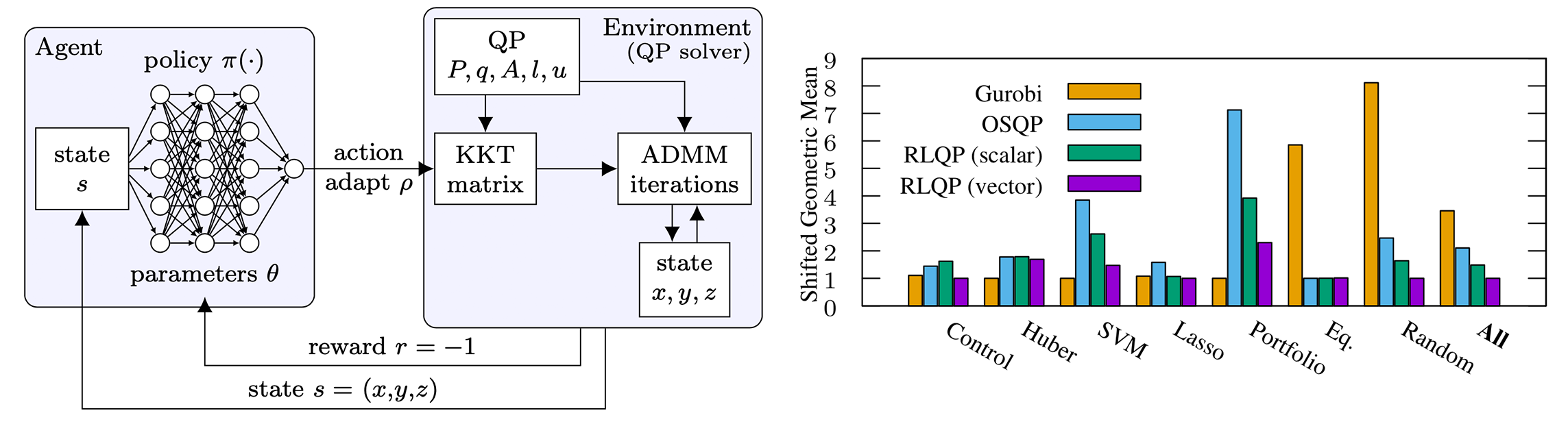# Accelerating Quadratic Optimizationwith Reinforcement Learning

##### Ken Goldberg
###### UC BerkeleyWe demonstrate reinforcement learning can significantly accelerate first-order optimization, outperforming state-of-the-art solvers by up to 3x. RLQP avoids suboptimal heuristics within solvers by tuning the internal parameters of the ADMM algorithm. By decomposing the policy as a multi-agent partially observed problem, RLQP adapts to unseen problem classes and to larger problems than seen during training.

## Video

Video will be posted alongside NeurIPS 2021, Dec 6 – 14.

## Summary

• Quadratic programming (QP) is a critical tool in robotics and finance. However, first-order solvers are slow for large problems requiring 1000s of iterations to converge.
• ADMM-based QP solvers are state-of-the-art at QPs optimization but these methods have numerous problem-specific ad-hoc heuristics that must be empirically tuned for good performance.
• In RLQP, we propose an RL policy to adaptively select the critical $$\rho$$ parameter in an open-source ADMM-based solver. Our policy is trained on a distibuion of randomly generated QPs.
• We extend Twin Delayed DDPG (TD3) to a multi-agent formulation in order to enable RLQP to generalize to novel out-of-distribution QPs at test time. Factorizing the agent enables evaluating with variable dimension observation and action spaces.
• Overall, RLQP is 4x faster than Gurobi and 3x faster than OSQP when trained over a benchmark set of QPs. Further speedups can be attained by speciaizing a policy for a single class of QPs. RLQP generalizes to challenging out-of-distribution QPs from the Netlib as well as the Maros and Meszaros problem sets.

## Abstract

First-order methods for quadratic optimization such as OSQP are widely used for large-scale machine learning and embedded optimal control, where many related problems must be rapidly solved. These methods face two persistent challenges: manual hyperparameter tuning and convergence time to high-accuracy solutions. To address these, we explore how Reinforcement Learning (RL) can learn a policy to tune parameters to accelerate convergence. In experiments with well-known QP benchmarks we find that our RL policy, RLQP, significantly outperforms state-of-the-art QP solvers by up to 3x. RLQP generalizes surprisingly well to previously unseen problems with varying dimension and structure from different applications, including the QPLIB, Netlib LP and Maros-Mészáros problems.

We consider optimizing quadratic programs (QPs) with $$n$$ variables and $$m$$ constraints of the form: $\begin{equation*} \begin{array}{ll} \mbox{minimize} & (1/2)x^TPx + q^Tx\\ \mbox{subject to} & l \le Ax \le u, \end{array} \end{equation*}$ With a positive semi-definite $$P \in \mathbb{S}_+$$, this QP represents a convex optimization problem.

These problems find frequent application to finance, machine learning and robotic control. For example, quadratic programming is critical to Model Predictive Control (MPC) for robotics. Given a problem with linear dynamics, the optimal solution is derived by solving a QP.

The current SOTA solver for QPs is the Operator Splitting Quadratic Program solver which is based on ADMM. OSQP first factorizes the KKT matrix derived from optimality conditions and then iteratively applying a series of updates scaled by the step-size parameter $$\rho$$. However, optimal choice of $$\rho$$ is difficult or impossible to determine. While some offline methods exist, these methods rely on solving expensive SDPs. Frequently, simple heuristics are used that attempt to balance the primal and dual residuals. For example, OSQP will set the $$\rho$$ vector to $$\rho^{(k+1)} \leftarrow \rho^{(k)} \sqrt{\|\xi_\mathrm{primal}\| / \|\xi_\mathrm{dual}\|}$$.

## Accelerating QP optimization with RLQP

In order to automatically select $$\rho$$, RLQP learns a policy to automatically adapt a single scalar $$\bar{\rho}$$ value to be used for all constraints. Integrating this policy into solvers is straightforward as the current heuristic in OSQP already generates a single scalar adaptation. This policy also can simply check that the proposed change to $$\bar\rho$$ is sufficiently small and avoid a costly matrix factorization.

In both handcrafted and RL cases, the policy is a function $$\pi : S_{\bar\rho} \rightarrow A_{\bar\rho}$$, where $$S_{\bar\rho} \in \mathbb{R}^2$$ are the primal and dual residuals stacked into a vector, $$A_{\bar\rho} \in \mathbb{R}$$ is the value to set to $$\bar\rho$$. The observation space and action space closely reflect what the current heuristic considers in QP solvers.

This simple scalar policy outperforms the handwritten heuristic in OSQP. However, this simple policy does not consider variations in how $$\rho$$ should be adapted across constraints. However, it is not clear how to craft a policy for a simple vectorized environment while supporting problems with a variable number of constraints. Ideally, a policy will consider an observation space of all residuals with an action space to predict all $$\rho$$ values at once. However, this policy would need to be trained for a particular number of constraints and would not adapt to arbitrary problems. The

Instead, we consider a reformulation of the vectorized environment as a multi-agent partially-observed MDP. Given a QP with $$m$$ constraints, we factorize the global policy into $$m$$ indepentent policies that consider observations for a single constraint and predict a single $$\rho_i$$ value. Therefore, the observation spaces and action spaces for these policies are fully indepentent from each other. We then share parameters for the policy across all constraints. Remarkably, we find the vector policy generalizes to problems with more constraints than seen during training.

## Citation

Jeffrey Ichnowski, Paras Jain, Bartolomeo Stellato, Goran Banjac, Michael Luo, Francesco Borrelli, Joseph E. Gonzalez, Ion Stoica, Ken Goldberg. Accelerating Quadratic Optimization with Reinforcement Learning.  Proc. Conference on Neural Information Processing Systems (NeurIPS), 2021.

 @article{ichnowski2021rlqp,   title={Accelerating Quadratic Optimization with Reinforcement Learning},   author={Jeffrey Ichnowski, Paras Jain, Bartolomeo Stellato,     and Goran Banjac, Michael Luo, Francesco Borrelli     and Joseph E. Gonzalez, Ion Stoica, Ken Goldberg},   journal={Proc. Conference on Neural Information Processing Systems (NeurIPS)},   year={2021} }